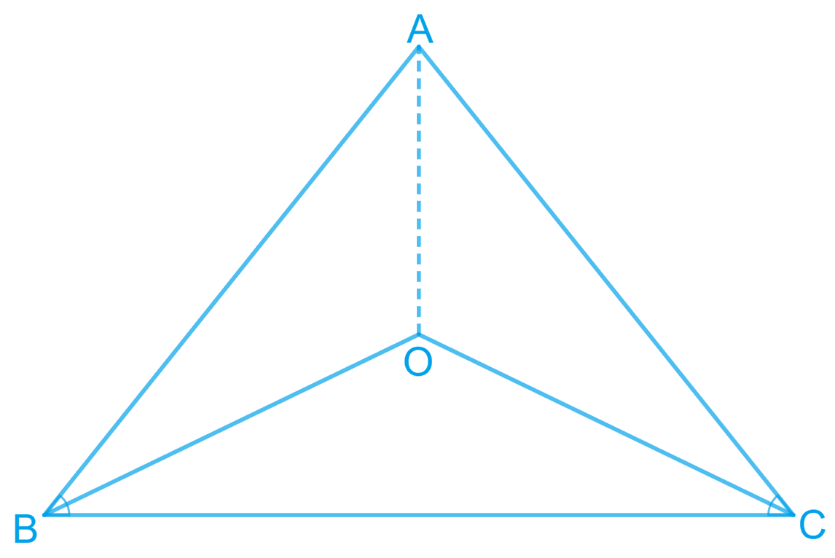# Ex.7.2 Q1 Triangles Solution - NCERT Maths Class 9

Go back to  'Ex.7.2'

## Question

In an isosceles triangle $$ABC$$, with

$$AB = AC$$, the bisectors of $$\angle B$$ and $$\angle C$$

intersect each other at $$O$$. Join $$A$$ to $$O$$.

Show that:

(i) $$OB = OC$$

(ii) $$AO$$ bisects $$\angle A$$

Video Solution
Triangles
Ex 7.2 | Question 1

## Text Solution

What is Known?

Triangle $$ABC$$ is isosceles in which $$AB=AC$$ also $$OB$$ and $$OC$$ are bisectors of angle $$B$$ and angle $$C$$

To prove:

(i) OB = OC

(ii) AO bisects ∠A

Reasoning:

As OB and OC are bisectors of angle B and angle C means half of angle B will be equal to half of angle c which will help us to conclude OB is equal to OC. Now We can show two triangles OAB and OAC congruent by using SSS congruency rule and then we can say corresponding parts of congruent triangles will be equal.Steps:

(i) It is given that in triangle $$ABC, AB = AC$$

\begin{align}& \angle ACB = \angle ABC \\&\left[ \begin{array} & \text{Angles opposite to equal } \\ \text{sides of a triangle are equal} \\ \end{array} \right]\\\\&\frac { 1 } { 2 } \angle ACB = \frac { 1 } { 2 } \angle ABC\end{align}

\begin{align} & \therefore OB=OC \\ & \left[ \begin{array} & \text{Sides}\,\,\text{opposite}\,\text{to}\,\text{equal}\,\text{angles}\, \\ \text{of}\,\text{a}\,\text{triangle}\,\text{are}\,\text{also}\,\text{equal} \\ \end{array} \right] \\ \end{align}

\begin{align} \rm (ii)\;\;&\text{In } \Delta OAB \text{ and } \Delta OAC, \\ & AO = AO\; (\text{Common}) \\ & AB = AC \;(\text{Given}) \\ & OB = OC\; (\text{Proved above}) \\ &\therefore \Delta OAB \cong \Delta OAC \\&\qquad(\text{By SSS congruence rule}) \end{align}

$\angle BAO = \angle CAO\;(CPCT)\\ \therefore AO \text{ bisects } \angle A$

Video Solution
Triangles
Ex 7.2 | Question 1

Learn from the best math teachers and top your exams

• Live one on one classroom and doubt clearing
• Practice worksheets in and after class for conceptual clarity
• Personalized curriculum to keep up with school